# 2021年中国高校大数据挑战赛A题完整版

wuchangjian2021-10-30 03:12:10编程学习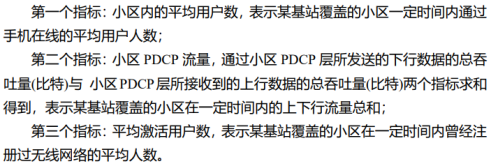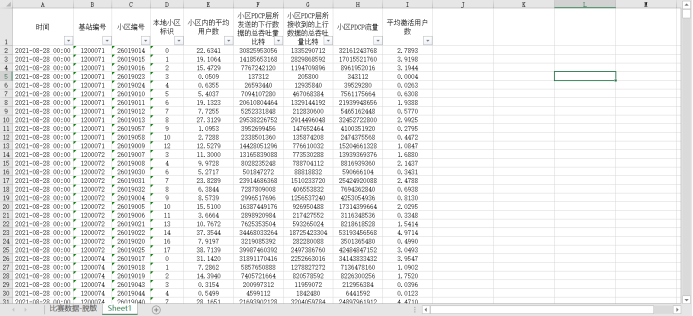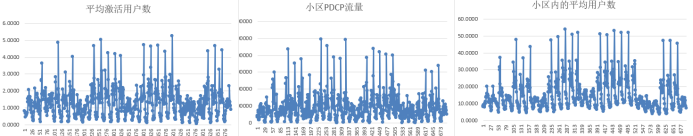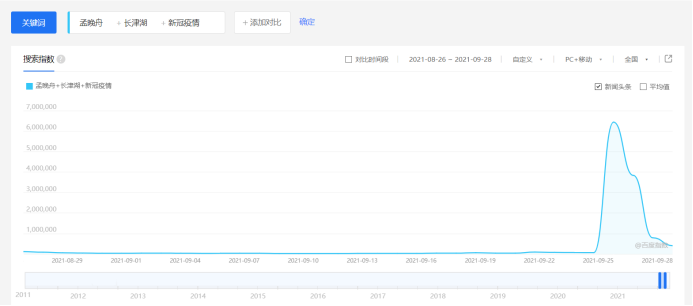``````import requests
word_url = 'http://index.baidu.com/api/SearchApi/thumbnail?area=0&word={}'
'Accept': 'application/json, text/plain, */*',
'Accept-Encoding': 'gzip, deflate',
'Accept-Language': 'zh-CN,zh;q=0.9',
'Cache-Control': 'no-cache',
'DNT': '1',
'Host': 'index.baidu.com',
'Pragma': 'no-cache',
'Proxy-Connection': 'keep-alive',
'Referer': 'http://index.baidu.com/v2/main/index.html',
'User-Agent': 'Mozilla/5.0 (Macintosh; Intel Mac OS X 10_13_6) AppleWebKit/537.36 (KHTML, like Gecko) Chrome/75.0.3770.90 Safari/537.36',
'X-Requested-With': 'XMLHttpRequest',
}

words = [[{"name": "猪肉", "wordType": 1}]]
keyword = str(words).replace("'", '"')
start='2010-12-27'
end='2021-10-10'
url = f'http://index.baidu.com/api/SearchApi/index?area=0&word={keyword}&area=0&startDate={start}&endDate={end}'#接口
data = resp.json().get('data')#读取后发现数据被加密，需要解码
user_indexes = data.get('userIndexes')
uniqid = data.get('uniqid')#读取uniqid参数
url = 'http://index.baidu.com/Interface/ptbk?uniqid={}'
ptbk = resp.json().get('data')#获取相应的json数据，也是解码
all_data = user_indexes.get('all').get('data')
pc_data = user_indexes.get('pc').get('data')
wise_data = user_indexes.get('wise').get('data')
#构建字典
n = list(ptbk)
a = {}
ln = int(len(n)/2)
start = n[ln:]
end = n[:ln]
for j,k in zip(start, end):
a.update({k: j})
#总指数=pc端搜索指数+移动端搜索指数
result1 = []
for j in all_data:
result1.append(a.get(j))#解码后为单个字符
result1 = ''.join(result1)#组合
result1 = result1.split(',')#以逗号切分
print('总指数')
print(result1)
#pc端搜索指数
result2 = []
for j in pc_data:
result2.append(a.get(j))#解码后为单个字符
result2 = ''.join(result2)#组合
result2 = result2.split(',')#以逗号切分
print('pc端搜索指数')
print(result2)
#移动端搜索指数
result3 = []
for j in wise_data:
result3.append(a.get(j))#解码后为单个字符
result3 = ''.join(result3)#组合
result3 = result3.split(',')#以逗号切分
print('移动端搜索指数')
print(result3)
``````

``````https://www.arcgis.com/apps/dashboards/index.html#/bda7594740fd40299423467b48e9ecf6
``````

``````https://ourworldindata.org/coronavirus#growth-of-cases-how-long-did-it-take-for-the-number-of-confirmed-cases-to-double
``````

``````http://www.hoonew.cn/newPneu_china/
``````

``````http://quote.eastmoney.com/sz300059.html?from=Sougou
``````

``````https://datacenter.jin10.com/
``````

``````https://d.qianzhan.com/
``````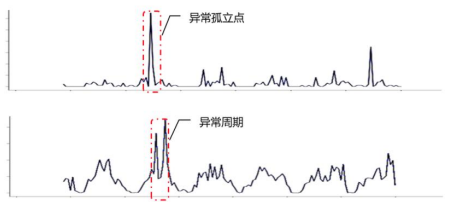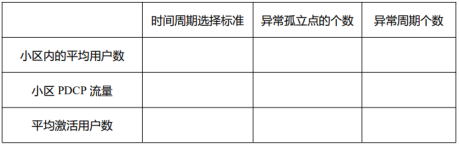``````function T_mean=period_mean_fft(data)
%该函数使用快速傅里叶变换FFT计算序列平均周期
%data：时间序列
%T_mean：返回快速傅里叶变换FFT计算出的序列平均周期
Y = fft(data);       %快速FFT变换
N = length(Y);    %FFT变换后数据长度
Y(1) = [];           %去掉Y的第一个数据，它是data所有数据的和
power = abs(Y(1:N/2)).^2;  %求功率谱
nyquist = 1/2;
freq = (1:N/2)/(N/2)*nyquist; %求频率
figure
plot(freq,power); grid on     %绘制功率谱图
xlabel('频率')
ylabel('功率')
title('功率谱图')
period = 1./freq;                %计算周期
figure
plot(period,power); grid on  %绘制周期－功率谱曲线
ylabel('功率')
xlabel('周期')
title('周期—功率谱图')
[mp,index] = max(power);       %求最高谱线所对应的下标
T_mean=period(index);            %由下标求出平均周期
``````

F值 = 精确率 * 召回率 * 2 / ( 精确率 + 召回率) （F 值即为精确率和召回率的调和平均值）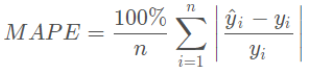### C语言知识6

1.%#X表示以十六进制形式输出，并附带前缀0X；C语言用变...

...

...

### 21 天好习惯”第一期－8

今天这道题可以用俩种解法： 一：...

#### 发表评论◎欢迎参与讨论，请在这里发表您的看法、交流您的观点。